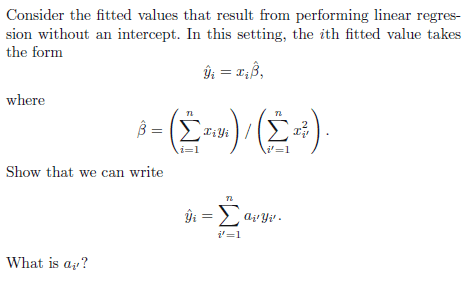# Linear/Cubic Regression

brojesus111

## Homework Statement

I collect a set of data (n = 100 observations) containing a single predictor and a quantitative response. I then fit a linear regression model to the data, as well as a separate cubic regression.

1) Suppose that the true relationship between X and Y is linear. Consider the training residual sum of squares (RSS) for the linear regression, and also the training RSS for the cubic regression. Would we expect one to be lower than the other, would we expect them to be the same, or is there not enough information to tell? Justify your answer.

2) Answer the above using test rather than training RSS.

3) Suppose that the true relationship between X and Y is not linear, but we don’t know how far it is from linear. Consider the training RSS for the linear regression, and also the training RSS for the cubic regression. Would we expect one to be lower than the other, would we expect them to be the same, or is there not enough information to tell? Justify your answer.

4) Answer the above with test rather than training RSS.

5)## The Attempt at a Solution

Attempt at 1: Not enough information since the training data could be wobbly, which in that case despite the true linear relationship, the cubic might fit better. But the training data could also be fairly linear, so the linear would be better and the cubic too wobbly.

Attempt at 2: In this case, the linear will be better since we are using the test RSS and if it is truly linear, then the linear regression should give a lower RSS since the fit will be better than the cubic.

Attempt at 3: Chances are that the cubic regression will provide the lower RSS. The linear will not provide a good fit for the non-linear relationship and even if the training data is less or more non-linear than the cubic regression, the cubic should provide the lower RSS since it should provide the better fit since it has more coefficients than the linear regression.

Attempt at 4: The cubic regression should give a lower RSS since it is not linear and our true relationship is not linear.

Attempt at 5: I'm assuming I have to solve for y_i from the ß^hat equation and then figure out what the a_i means given that, but I'm stuck on how to solve for y_i.

Any tips, help, corrections, etc. would be great.

## Answers and Replies

Staff Emeritus
Try again. Hint: A linear relation ax+b is a special case of a cubic, represented as a cubic as 0x3+0x2+ax+b.

•1 person
brojesus111
Try again. Hint: A linear relation ax+b is a special case of a cubic, represented as a cubic as 0x3+0x2+ax+b.

Ok, so this is my revised answer:

1) The cubic will give the lower RSS since adding another variable to a least square equation must allow us to fit the training data better.

2) I think my answer to this one is right. The RSS for the linear should still provide the lower RSS since the cubic would be too wobbly.

3) Same reasoning as #1. Since we have more variables, we should be able to fit the training observations better.

4) I'm not completely sure on this one, but I think the answer is cubic since the true linear relationship is non-linear.

5) Still stuck.

brojesus111
Ok, so I have this for 5 now.

I replaced the beta in the original y-hat equation to get:

y-hat_i = x_i [sum{i=1,n} x_i y_i]/[sum{i'=1,n} x_i' ^2]
y-hat_i = sum{i'=1,n} f(x_i,x_i') y_i

So this means that a_i' is f(x_i, x_i').

Is this correct? The only thing that looks wrong is that I end up with y_i instead of y_i' in the end.

Last edited:
brojesus111
Realized my answer for #4 is wrong. We don't have enough information for that one.

Staff Emeritus
With your last post, everything looks good except for your work on #5.

Regarding #2, I'm not thrilled with the term "wobbly". It's a bit too, well, wobbly. If the relationship truly is linear, the linear part of the cubic will fit the signal. The quadratic and cubic terms will fit whatever is left after you remove the signal.
Hint: What's left after you remove the signal?

Another issue (again on #2): Suppose some of your test data lies outside the domain of the training data (i.e., you're extrapolating rather than interpolating). What happens to that cubic expression when applied outside the domain of the training data?

brojesus111
With your last post, everything looks good except for your work on #5.

Regarding #2, I'm not thrilled with the term "wobbly". It's a bit too, well, wobbly. If the relationship truly is linear, the linear part of the cubic will fit the signal. The quadratic and cubic terms will fit whatever is left after you remove the signal.
Hint: What's left after you remove the signal?

Another issue (again on #2): Suppose some of your test data lies outside the domain of the training data (i.e., you're extrapolating rather than interpolating). What happens to that cubic expression when applied outside the domain of the training data?

For #2, can I just say since that we explicitly know that the true relationship is linear, the linear model should minimize the test MSE?

For #5, can I do this?

I will give the summations their own variables.

y-hat_i = x_i beta
beta = [sum{f=1,n} x_f y_f ]/[sum{g=1,n} x_g ^2]
y-hat_i = x_i [sum{f=1,n} x_f y_f]/[sum{g=1,n} x_g ^2]

Since the summation of x_k is just a constant, we can rewrite it as:

y-hat_i = sum{f=1,n} f(x_i,x_f) y_f
Then substitute f=i' and we get our answer.

Staff Emeritus
What is your f(x_i,x_f)? You haven't defined it.

brojesus111
What is your f(x_i,x_f)? You haven't defined it.

It's x_i [sum{f=1,n} x_f]/[sum{g=1,n} x_g ^2]

Staff Emeritus